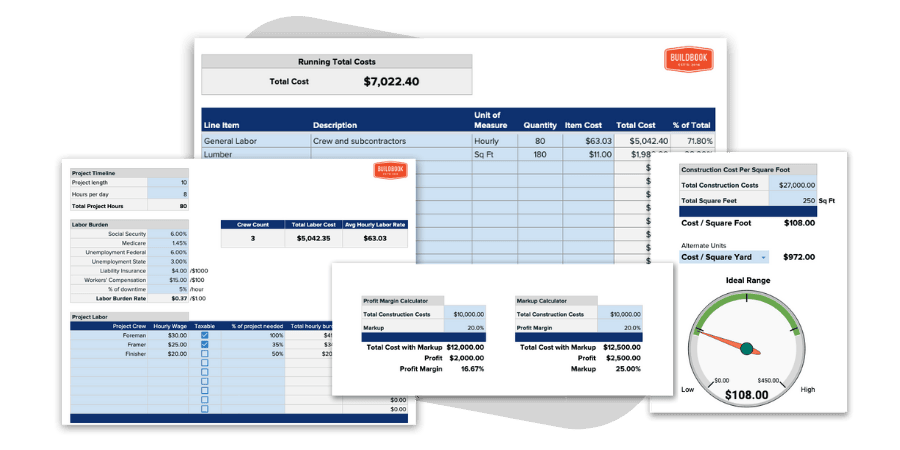# Construction CostsCalculate your total construction costs based on line items, rates, and quantities

## Want an even better construction cost calculator with more estimating tools?

### Download our free construction calculator collection!

This collection of construction calculators is 100% free to download, copy, and use as your own. You're free to modify and/or adjust any calculator to best fit the needs of you or your business.This Construction Cost Calculator is designed to help you itemize the rate and quantity of each individual element to estimate the total cost of construction project.

## How to accurately calculate construction costs?

Depending on the type of project, estimating the costs of construction can either be incredibly simple or extremely complex. Regardless, creating an accurate estimate is essential to ensuring that the project is both profitable for the contractor and affordable for the client. A good construction cost estimate will account for every element of a project, including room for contingencies, financing costs, profit margin, variances, and other less obvious or unforeseen expenses. Changes are almost a guarantee in construction, so it's to everyone's benefit not to underestimate or overlook any element of a project.

## The primary construction cost categories

In order for your estimate to be as thorough and accurate as possible, it's best to at least consider every potential category when calculating the costs for a project. Each category or element may have additional considerations that could impact the cost so it may not be as simple as associating a single cost to any given cost category.

As you're calculating construction costs, consider each of the following potential cost categories:

• Site Preparation - Excavation, tear-downs, on-site rentals, disposal, etc.
• Material costs - Hardware, lumber, concrete, sheetrock, trim, flooring, etc.
• Labor costs - Labor burden, downtime, overtime, etc.
• Subcontractor costs - HVAC, plumbers, designers, landscapers, etc.
• Equipment costs - Heavy equipment fees, rentals, gas, power, etc.
• Permits and fees - Inspections, approvals, local permits, etc.
• Client Selections - Appliances, countertops, fixtures, etc.
• Indirect expenses - Administration costs, travel, delivery fees, etc.
• Capital costs - Loan interest, payment processing fee, taxes, etc.
• Profit Margin - Labor markup, material markup, general profit, etc.
• Contingencies - Seasonal challenges, client delays, cost escalations, wastage, etc.

Based on the location and/or type of the construction project, there may be other cost categories you will need to consider, or additional elements within the categories above.

## How to calculate labor cost in construction?

It is important to consider all of your additional costs when calculating your actual cost of labor. Failing to factor in the additional costs can have a large negative impact on the profit of your project.

The formula for calculating the construction labor cost for each employee is: (Hourly Wage + Hourly Labor Burden) * Hours Worked/Needed

To learn how to calculate your labor burden to determine your actual hourly labor cost, check out our construction labor cost calculator.

## How to calculate construction cost per square feet

Since the cost of construction is often measured and evaluated by its cost per square foot, you may want to determine this number for your construction project.

Once your total construction cost is determined, calculating the cost per square foot is as simple as dividing the cost by the total square footage of the project.

The formula is as follows: Total Cost of Construction / Total Square Feet = Construction Cost Per Square Foot

To help calculate the total square feet of your project, use our construction square footage calculator.